Get instant live expert help with Excel or Google Sheets“My Excelchat expert helped me in less than 20 minutes, saving me what would have been 5 hours of work!”

#### Post your problem and you’ll get expert help in seconds.

Your message must be at least 40 characters
Our professional experts are available now. Your privacy is guaranteed.

# How to Count Colored or Highlighted Cells in Excel

The COUNT function in Excel counts cells containing numbers in Excel. You cannot count colored or highlighted cells with the COUNT function. But you can follow a few workarounds to count colored cells in Excel. In this tutorial, you will learn how to count colored cells in Excel.

In excel, you can count highlighted cells using the following workarounds:

1. Applying SUBTOTAL and filtering the data
2. Using the COUNT and GET.CELL function
3. Using VBA

### Using Subtotal and Filter functions

You can count highlighted cells in Excel by subtotaling the visible cells and applying a filter based on colors.

In this example, you have the sales record for eight salespersons for the month of July. The rows containing salespersons having sales less than \$7000 is highlighted in red, the other cells with the salespersons having a bonus is highlighted in green. To calculate the number of salespersons highlighted in red:

• Select the cell C10.
• Assign the formula `=``SUBTOTAL(102, C2:C9)`. The first argument 102 counts the visible cells in the specified range.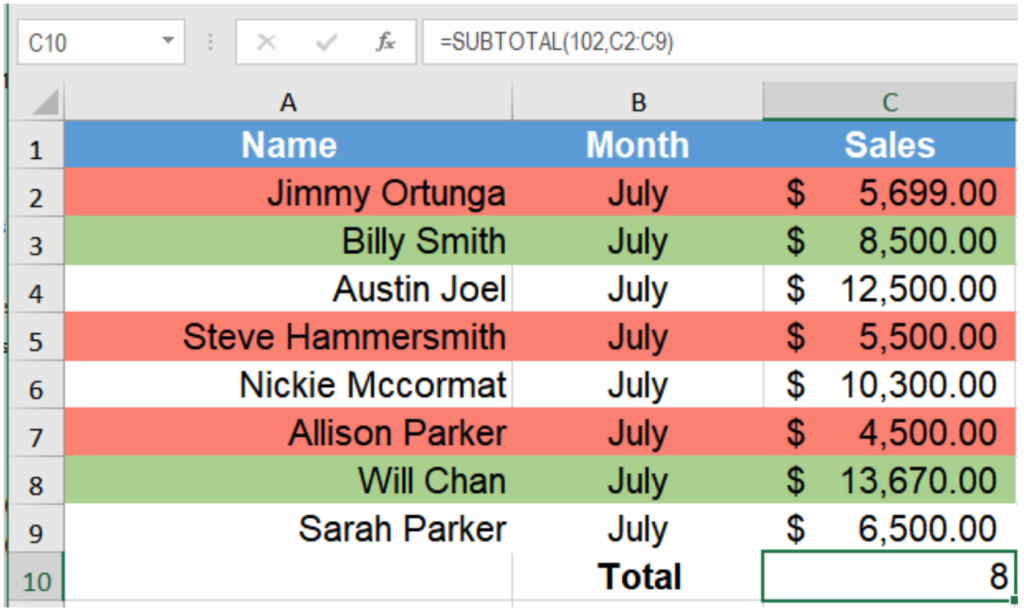• Select cells A1:C9 by clicking on cell A1 and dragging it till C9 with your mouse.
• Go to Data > Sort & Filter > Filter.• Click on the filter drop downs in C1.
• Go to Filter by Color and select the color Red to find out the salespersons highlighted in red.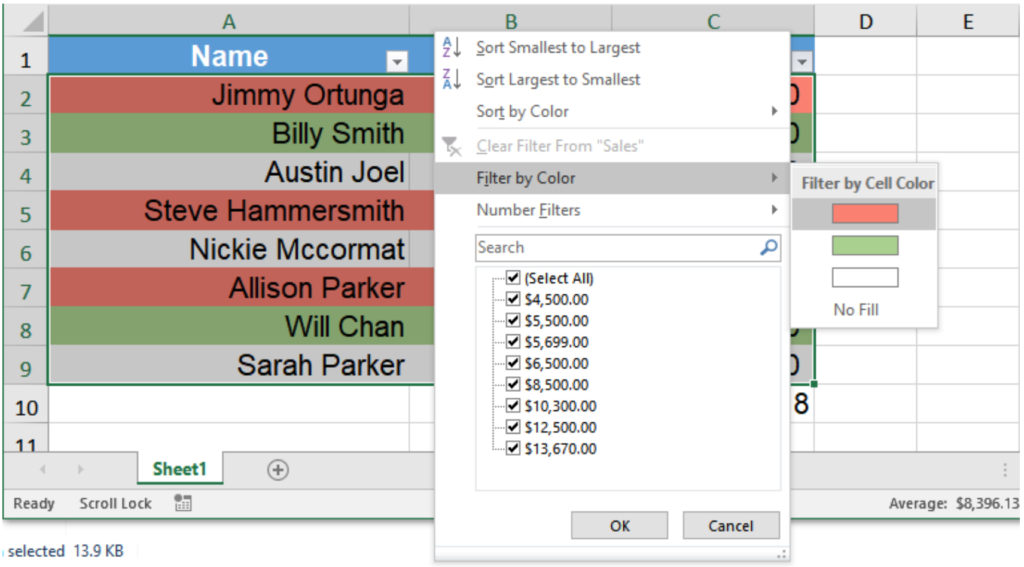This will output change the subtotal value to x, which is the number of salespersons having sales less than \$7000.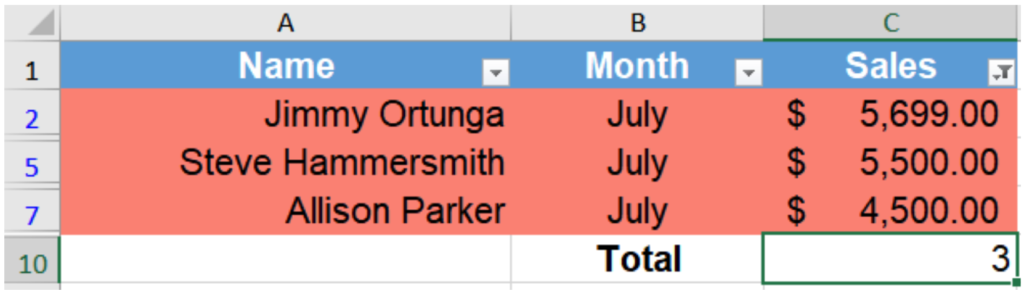### Using COUNTIF and GET.CELL functions

You can use GET.CELL with named ranges to count colored cells in Excel. GET.CELL is an old Macro4 function and does not work with regular functions. However, it still works with named ranges. To count colored cells with GET.CELL, you need to extract the color codes with GET.CELL and count them to find out the number of cells highlighted in the same color. To count cells using GET.CELL and COUNTIF:

• Go to Formulas > Define Name.• In the dialogue box that pops up, set name as ColorCount, scope as workbook and Refers to as `=GET.CELL(38, Sheet1!C2).`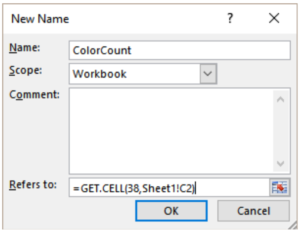• Assign the formula =ColorCount to cell D2 and drag it till D9 with your mouse.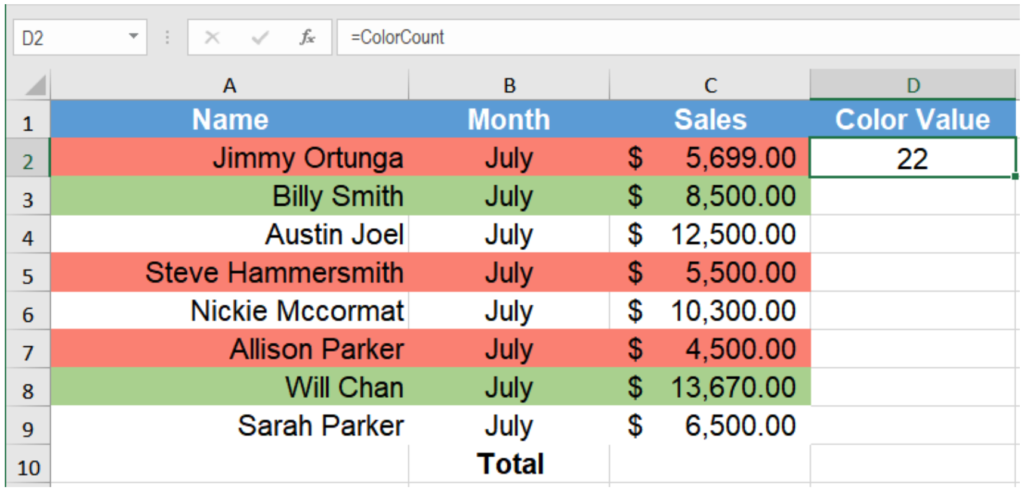• This would return a number based on the background color. If there is no background color, Excel would return 0. Otherwise all cells with the same background color return the same number.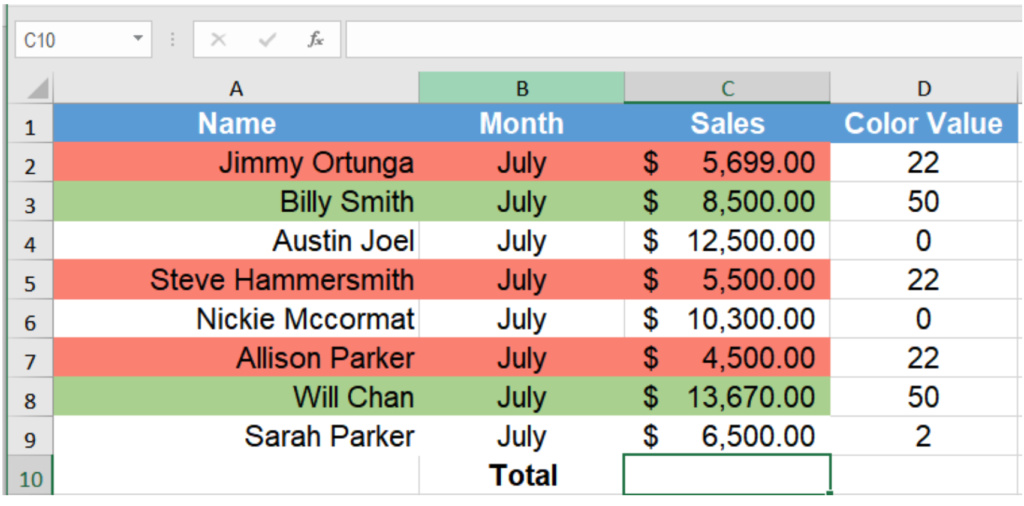• From column D, look at the code for the color red. In this case it is 22.
• Select cell D10 and assign the formula `= COUNTIF(\$D:\$D, 22).`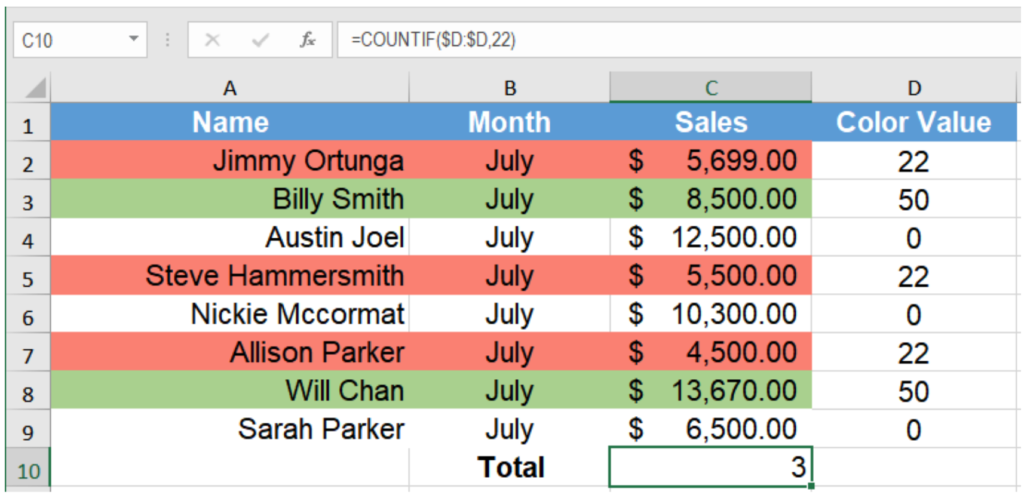This will count the cells colored in red to find the number of salespersons with sales less than \$7000 and return the number 3.

### Using VBA

You can also create a custom function with VBA to count highlighted cells in Excel. To do that you need to create a custom function using VBA that works like a COUNTIF function and returns the number of cells for the same color.

You will follow the syntax: =CountFunction(CountColor, CountRange) and use it like other regular functions.Here CountColor is the color for which you want to count the cells. CountRange is the range in which you want to count the cells with the specified background color.

To count the cells highlighted in red, follow the steps below:

• Hold down the ALT + F11 keys, and it opens the Microsoft Visual Basic for Applications window.
• Click Insert > Module.• Paste the following code in the Module Window.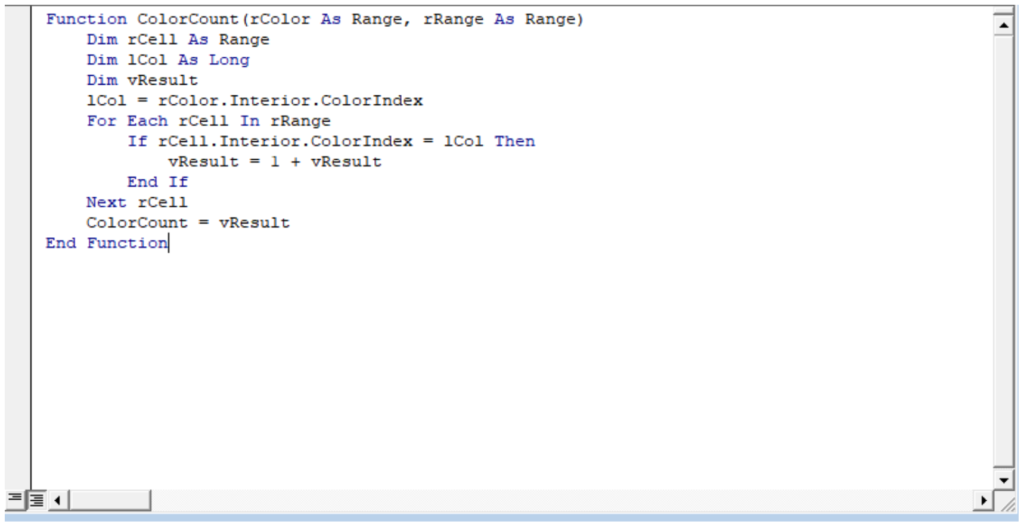• In this code, you are defining a function with two arguments rColor and rRange. You are going to save the value of the background color of A2 in lCol. Then you are going to run a FOR loop where, if the cell’s background color matches the color in lCol, you increment vResult. This function returns the value of vResult, which is the count of the cells having the same background color.
• Then save the code, and apply the following formula to cell C10:

`=ColorCount(A2, C2:C10).`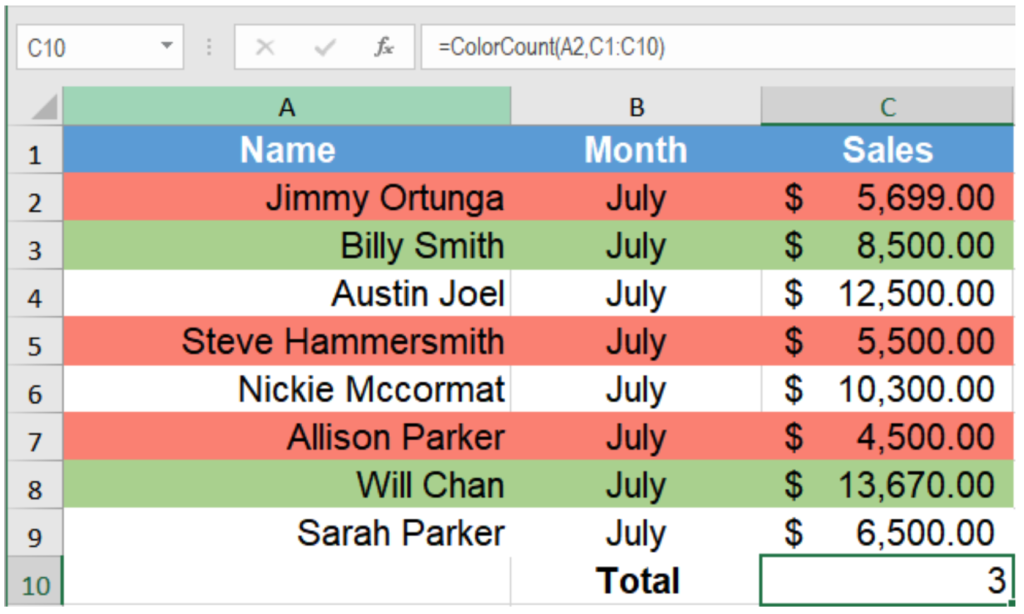• Here A2 is the cell with the background color red you want to count. C2:C10 is the cell range that you want to count.

This will return the number of salespersons with sales less than \$7000 in the month of July which is 3.

### Did this post not answer your question? Get a solution from connecting with the expert.Another blog reader asked this question today on Excelchat:
Solution examplesI have a column of percentages (11 in a column). I want to have a percentage at the bottom of the column that tells me how many of the entries are = to 100%.
Solved by X. E. in 20 minsI need a formula to count the number of rows that contain at least one of two possible phrases, but not give me the total number of instances that both phrases occur overall.
Solved by E. B. in 33 minsIs it possible to have a function (like a COUNTIF) that will count sells that meet specific criteria, but the range is limited to the 10 cells in the range that immediately precede a blank cell? So for example, the general range is A1:Z1, but I want the range to be specific to the last ten cells immediately prior to the first blank cell in the general range. Thank you.
Solved by O. F. in 26 minsNeed help putting together formula where it counts the number of sales but only if the average annual premium is above \$360. So a sales = Sum(N:2:N:48)/360....but not greater than COUNTIF(N:2:N:48) ""
Solved by O. J. in 43 minsI used the formula =COUNTIF for cells to define adding the number of cells that have numbers totaling 0 or above. However, When I try using it in another set of columns it is not doing what I need it to. Now, the column that is not working has both colored cells and formula'd numbers within the cells. I dont understand what I am doing wrong, please help!
Solved by C. H. in 20 mins## Subscribe to Excelchat.coAnother blog reader asked this question today on Excelchat: